Common Probability Distributions | IFT World
IFT Notes for Level I CFA® Program
IFT Notes for Level I CFA® Program

# Part 3

## 5. Applications of the Normal Distribution

Shortfall risk

Shortfall risk is the risk that portfolio’s return will fall below a specified minimum level of return over a given period of time.

Safety first ratio

Safety first ratio is used to measure shortfall risk. It is calculated as: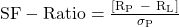where:

Rp = Expected portfolio return

RL = Threshold level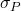= Standard deviation of portfolio returns

The portfolio with the highest SF-Ratio is preferred, as it has the lowest probability of falling below the target return.

Example

An investor is considering two portfolios A and B. Portfolio A has an expected return of 10% and a standard deviation of 2%. Portfolio B has an expected return of 15% and a standard deviation of 10%. The minimum acceptable return for the investor is 8%. According to Roy’s safety first criteria, which portfolio should the investor select?

Solution: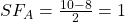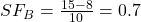Since A has a higher safety first ratio, the investor should select portfolio A.

Roy’s safety first criteria

It states that an optimal portfolio minimizes the probability that the actual portfolio return will fall below the target return.

## 6. Lognormal Distribution and Continuous Compounding

### 6.1 The Lognormal Distribution

If x is a random variable that is normally distributed, then to create a lognormal distribution of x we take ex and plot the values on a graph.

The properties of a lognormal distribution are:

• It cannot be negative.
• The upper end of its range extends to infinity.
• It is positively skewed.

Instructor’s Note: A normal distribution is more suitable as a model for returns than for asset prices, because returns generally vary about the mean, with a high probability of returns being close to the mean.

However, asset prices do not vary equally about a mean price, since the probability of extreme changes in price decreases as the price approaches zero. This means asset prices will not form a symmetrical graph like that of the normal distribution. Instead, asset prices follow a lognormal distribution, which is skewed to the right and cannot be negative.

### 6.2 Continuously Compounded Rates of Return

Discretely compounded rates of returns have defined compounding periods such as quarterly, monthly etc. As we decrease the length of the compounding period, the effective annual rate rises. For continuous compounding, the EAR is given by:

EAR = er – 1

If we are given the holding period return over any time period, we can calculate the equivalent continuously compounded rate of return for that period as:

r = ln (HPR +1)

Example

If the holding period return of a stock was 10% for a period of one year. What is the equivalent continuously compounded rate of return for the year?

Solution:

r = ln (0.1 +1) = 0.0953 = 9.53%

## 7. Student’s t-, Chi-Square, And F-Distributions

The Student’s t, chi-square, and F-distributions are mostly used to support statistical analyses, such as sampling, testing the statistical significance of estimated model parameters, or hypothesis testing.

### 7.1 Student’s t-Distribution

The properties of a Student’s t-distribution are:

• It is symmetrical, bell-shaped and similar to a normal distribution.
• It has a lower peak and fatter tails as compared to a normal distribution.
• It is defined by a single parameter, degrees of freedom (df) = n – 1.
• As the df increase the t-distribution approaches the standard normal distribution.

##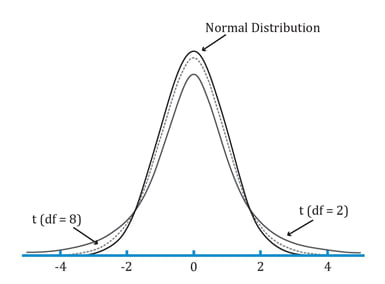### 7.2 Chi-Square and F-Distribution

The properties of the Chi-square distribution are:

• It is asymmetrical and is defined by a single parameter, degrees of freedom (df) = n – 1
• With k degrees of freedom, the distribution is the sum of the squares of k independent standard normally distributed random variables. Therefore, the distribution does not take on negative values.
• A different distribution exists for each value of df, n-1.
• As the df increase the shape of the distribution becomes more similar to a bell curve.

##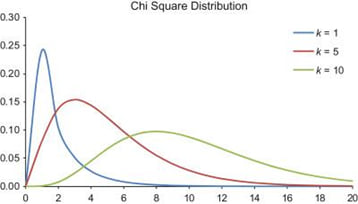The properties of the F-distribution are:

• It is asymmetrical and defined by two parameters, degrees of freedom of the numerator (df1) and degrees of freedom of the denominator (df2).
• The distribution does not take on negative values.
• As both the numerator (df1) and the denominator (df2) degrees of freedom increase, the distribution becomes more similar to a bell curve.

##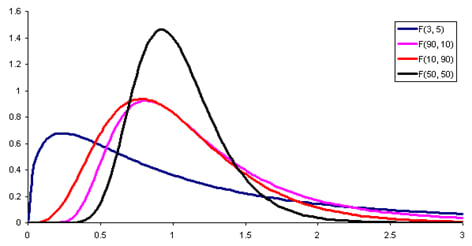The relationship between the chi-square and F-distributions is as follows: If  is one chi-square random variable with m degrees of freedom and  is another chi-square random variable with n degrees of freedom, then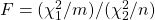follows an F-distribution with m numerator and n denominator degrees of freedom.

## 8. Monte Carlo Simulation

Monte Carlo simulation is a computer simulation used to simulate possible security prices based on risk factors. As input, it uses randomly generated values for risk factors based on their assumed distributions. It processes this information as per the specified model and runs thousands of iterations. Then it gives the distribution of the expected value of the security as output.

Major applications include:

• financial planning
• developing VAR estimates
• valuing complex securities

Limitations include:

• It is fairly complex and will provide answers that are no better than the assumptions.
• Simulation is not an analytical method but a statistical one.